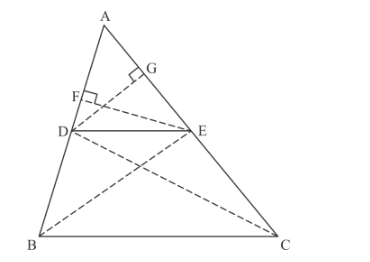# If a line is drawn parallel to one side of a triangle`
Question:

If a line is drawn parallel to one side of a triangle to intersect the other two sides in distinct points, prove that the other two sides are divided in the same ratio.

Solution:

Given that ain which a line parallel to BC is drawn which meet the other two sides at the point D and E, then we have to prove that

$\frac{\mathrm{AD}}{\mathrm{DB}}=\frac{\mathrm{AE}}{\mathrm{EC}}$

We have the following diagram with some additional construction.In the above figure, we can see that EF is perpendicular to AB. Therefore EF is the height of the.

Now we are finding the area ofand area ofArea of $\triangle \mathrm{ADE}=\frac{1}{2}($ base $\times$ hight $)$

$=\frac{1}{2} \mathrm{AD} \cdot \mathrm{EF}$..............(1)

Area of $\triangle \mathrm{DBE}=\frac{1}{2} \mathrm{DB} . \mathrm{EF}$............(2)

Now take the ratio of the two equation, we have

$\frac{\text { Area of } \triangle \mathrm{ADE}}{\text { Area of } \triangle \mathrm{DBE}}=\frac{\frac{1}{2} \mathrm{AD} . \mathrm{EF}}{\frac{1}{2} \mathrm{DB} \cdot \mathrm{EF}}$

$=\frac{\mathrm{AD}}{\mathrm{DB}}$............(3)

Similarly, In $\triangle \mathrm{ADE}$ and $\triangle \mathrm{DEC}$, we have

$\frac{\text { Area of } \triangle \mathrm{ADE}}{\text { Area of } \triangle \mathrm{DEC}}=\frac{\frac{1}{2} \mathrm{AE.DG}}{\frac{1}{2} \mathrm{EC.DG}}$

$=\frac{\mathrm{AE}}{\mathrm{EC}}$...............(4)

But, the area of triangle DBE and triangle DEC are same, therefore equation (4) can be written as

$\frac{\text { Area of } \triangle \mathrm{ADE}}{\text { Area of } \triangle \mathrm{DBE}}=\frac{\frac{1}{2} \mathrm{AE} \cdot \mathrm{DG}}{\frac{1}{2} \mathrm{EC} \cdot \mathrm{DG}}$

$=$..............(5)

From equation (3) and equation (5), we get

$\frac{\mathrm{AD}}{\mathrm{DB}}=\frac{\mathrm{AE}}{\mathrm{EC}}$

Hence, $\frac{\mathrm{AD}}{\mathrm{DB}}=\frac{\mathrm{AE}}{\mathrm{EC}}$